Assignment Operators in python

Assignment operators are used to assigning values to variables.

That is to store values in variables we use = assignment operator.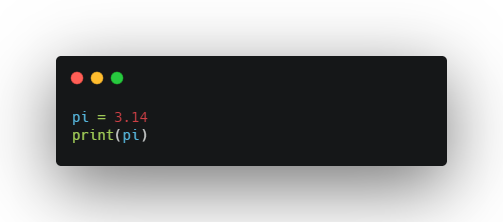Output:

3.14

OK, now comes the real fun. Have ever been tired to use x = x + 5, where we type the variable x twice? There’s actually a shortcut for this called Augmented assignment operators.

Augmented assignment operators can be used as a replacement as follows:

x += 3	   --->	   x = x + 3
x -= 3	   --->	   x = x - 3
x *= 3	   --->	   x = x * 3
x /= 3	   --->	   x = x / 3
x %= 3	   --->	   x = x % 3
x //= 3	   --->	   x = x // 3
x **= 3	   --->	   x = x ** 3
x &= 3	   --->	   x = x & 3
x |= 3	   --->	   x = x | 3
x ^= 3	   --->	   x = x ^ 3
x >>= 3	   --->	   x = x >> 3
x <<= 3	   --->	   x = x << 3

Here’s the Code and Output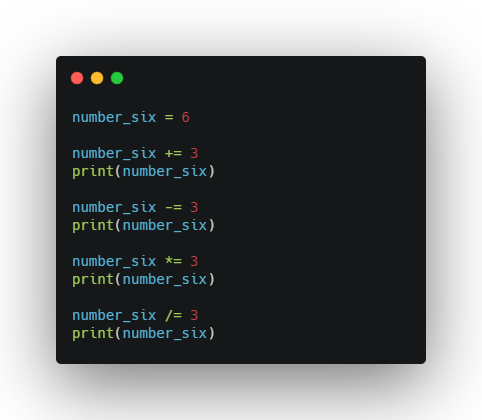9
6
18
6.0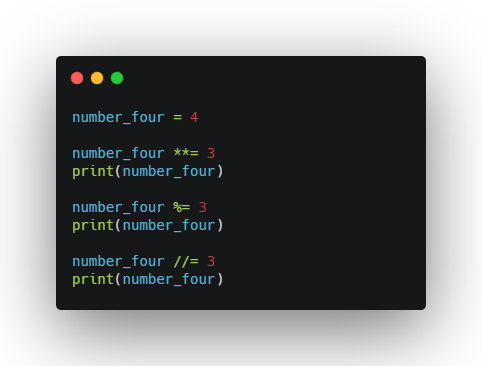64
1
0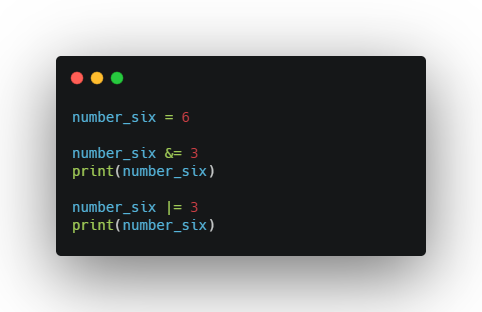2
3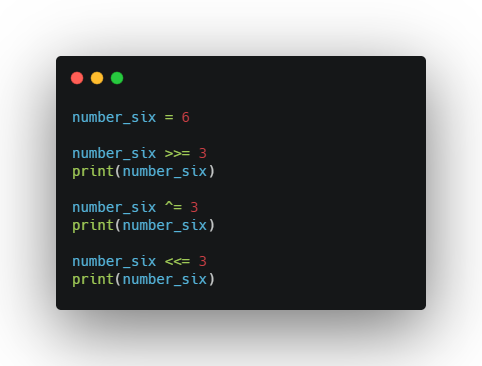0
3
24

Quick Note: The code snippets reuses the same variable to assign with different arithmetic operations / bitwise operations / shift operations.

So, while coding makes sure you practice to use print statements after each operation.

Code along and have fun ;)Aswin Barath

My name is Aswin Barath. I’m a technical blogger and budding software engineer